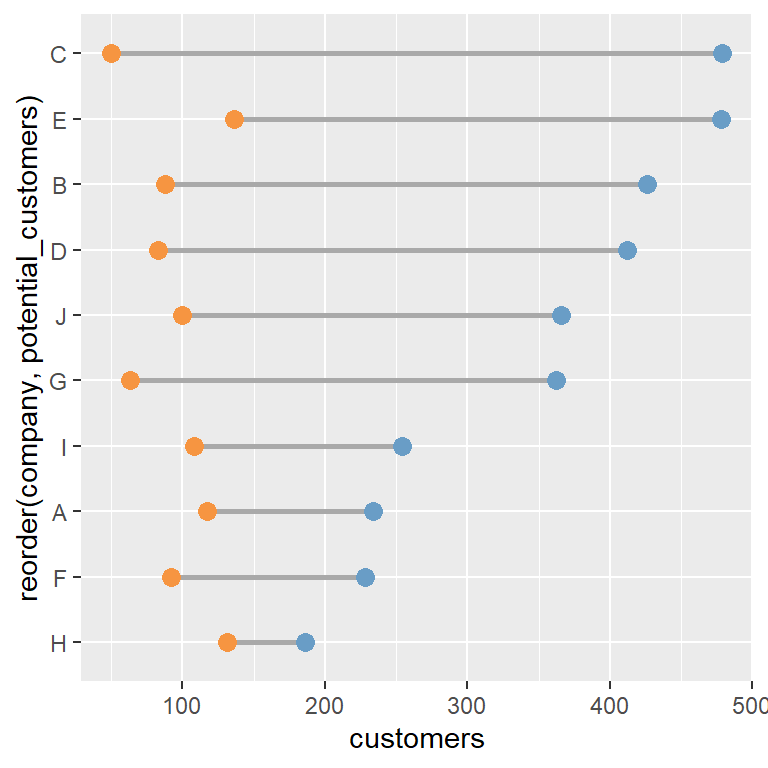# Dumbbell plot in ggplot2

A dumbbell plot is a type of a dot plot where the two points for each group are connected with straight lines. This chart can be used to display the changes of a variable between two different points of time or to show the range of a variable across several groups, such as the growth of population at two different years across several countries or the difference between the current and potential customers of different companies.

The applications of this type of chart are wide, as it can be used to analyze the distribution of the groups based on their range, to create a ranking or even to analyze correlations across groups.

## Sample data sets

Depending on your data you can create a dumbbell plot in a different way. Sometimes it is easier just to transform your data to a more suitable format, but in this tutorial we are going to review how to create a dumbbell chart in ggplot2 both with long (`df`) and wide (`df2`) data frames, so two sample data frames are provided.

We encourage you to always use long format when working with ggplot2, as it was designed for it.

### Wide data frame

The following data frame contains the current and potential customers of different companies. Note that each row represents a different company.

``````# Seed
set.seed(1)

# Data
customers <- sample(50:150, 10)
potential_customers <- sample(150:500, 10)
company <- LETTERS[1:10]

# Data frame
df <- data.frame(company = company,
x = customers,
y = potential_customers)``````

### Long data frame

The `melt` function from `reshape` allows converting a wide data frame into long format. This format displays the variables as subgroups of the main groups, so each group will have as many rows as variables and the numerical values will all be in the same column instead of splited into different columns.

``````library(reshape)

# Seed
set.seed(1)

# Data
customers <- sample(50:150, 10)
potential_customers <- sample(150:500, 10)
company <- LETTERS[1:10]

# Data frame
df2 <- data.frame(company = company,
customers = customers,
potential_customers = potential_customers)

# Long, ordered data frame
df2 <- melt(df2, id.vars = "company")
df2 <- df2[order(df2\$company), ]``````

## Dumbbell chart with `geom_segment` and `geom_point`

Depending on your data frame you will need a different approach to create a dumbbell plot in ggplot2.

Option 1: wide data frame

If you are working with a wide data frame you can create a dumbbell chart by adding the straight lines with `geom_segment`, specifying the start and end for both axis. Then, you will need to use the `geom_point` function twice to add the points. Note that you won’t be able to add a legend in a straightforward way using this format.

``````# install.packages("ggplot2")
library(ggplot2)

ggplot(df) +
geom_segment(aes(x = customers, xend = potential_customers,
y = company, yend = company)) +
geom_point(aes(x = customers, y = company), size = 3) +
geom_point(aes(x = potential_customers, y = company), size = 3)``````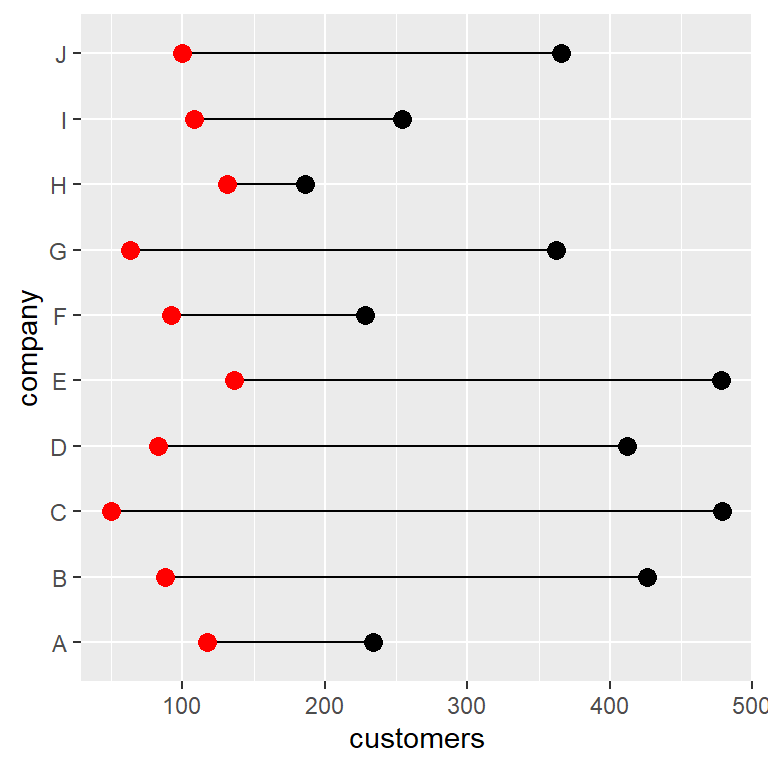Option 2: long data frame

The long data frame format is the most recommended to create this type of visualization. You just need to input the names of the columns that represents values and groups to `x` and `y` inside `aes` and use the `geom_line` and `geom_point` functions. If you want to display a legend for the points you just need to input the name of the column representing subgroups to `color` inside `aes`. Recall that you can customize the legend position with `legend.position`.

``````# install.packages("ggplot2")
library(ggplot2)

ggplot(df2, aes(x = value, y = company)) +
geom_line() +
geom_point(aes(color = variable), size = 3) +
theme(legend.position = "bottom")``````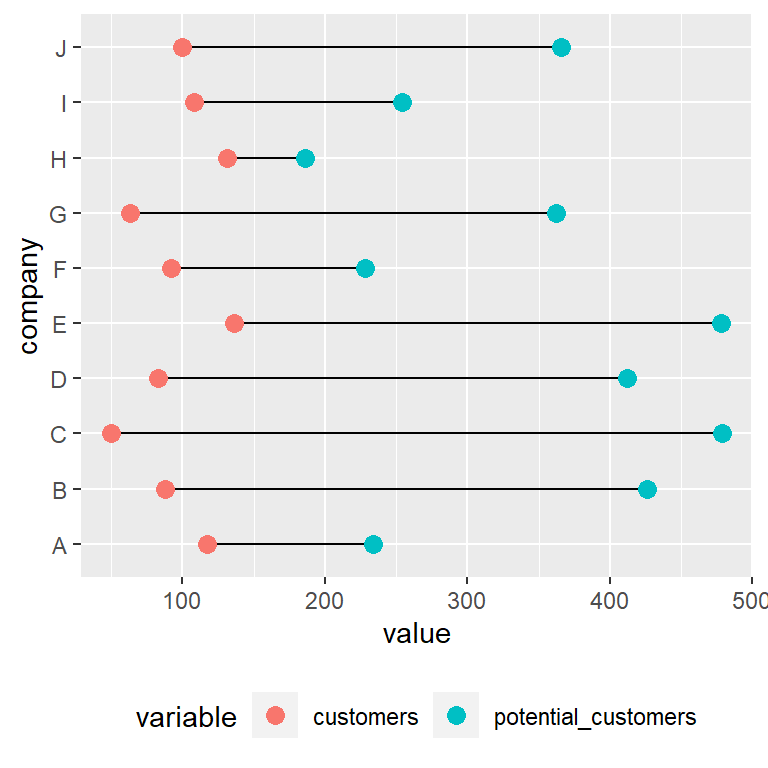Customizing the color of the points

When working with a long data frame you can override the default colors of the points with a scale color function, such as `scale_color_manual` or `scale_color_brewer`.

``````# install.packages("ggplot2")
library(ggplot2)

ggplot(df2, aes(x = value, y = company)) +
geom_line() +
geom_point(aes(color = variable), size = 3) +
scale_color_brewer(palette = "Set1", direction = -1) +
theme(legend.position = "bottom")``````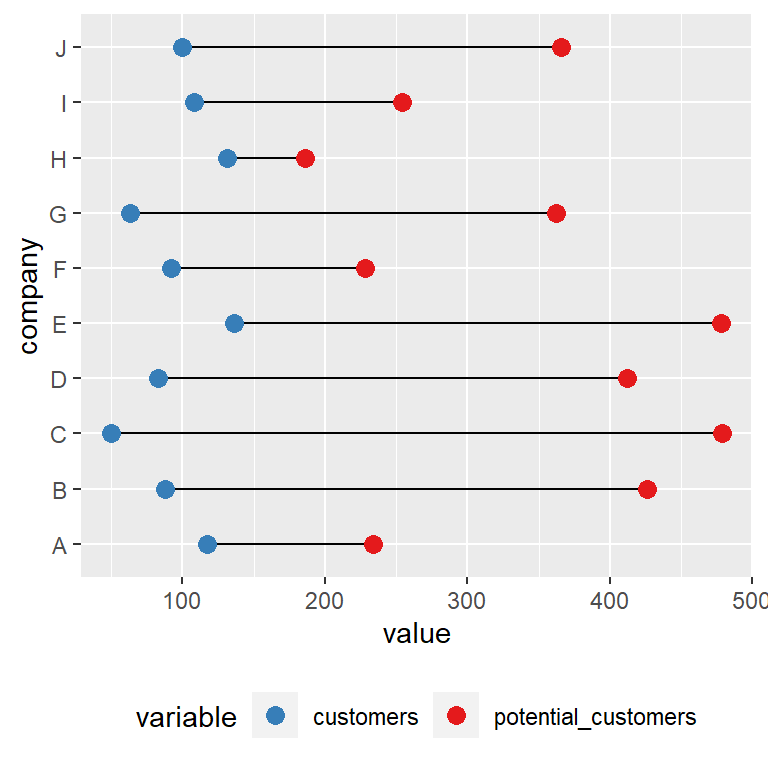## Dumbbell plot with `ggalt` and `geom_dumbbell`

An alternative to `geom_segment` and `geom_point` to create a dumbbell plot is the `geom_dumbbell` function from `ggalt` package. Using this function is straightforward, but the input data frame must be in wide format.

The function requires the groups (`x` or `y`), the start value (`x` or `y`) and the end positions (`xend` or `yend`). In addition, the function provides several arguments to customize the width and color of the lines and markers.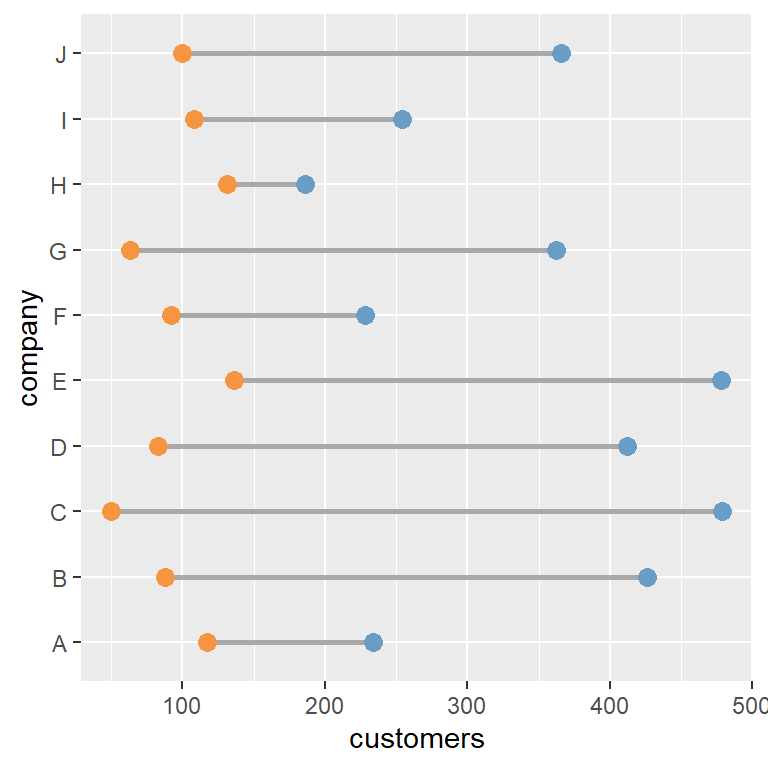``````# install.packages("ggplot2")
# install.packages("ggalt")
library(ggplot2)
library(ggalt)

ggplot(df, aes(y = company, x = customers, xend = potential_customers)) +
geom_dumbbell(color = "darkgray",  # Color of the line
size = 1,            # Line width
dot_guide = FALSE,   # Whether to add a guide from origin to X or not
size_x = 3,          # Size of the X point
size_xend = 3,       # Size of the X end point
colour_x = "#F69541",    # Color of the X point
colour_xend = "#699DC6") # Color of the X end point``````

The main drawback of this function is that if you want to add a legend you will need to transform the data to long format and add the points again as we did in the previous example.

## Ordered dumbbell plot

A dumbbell plot can be ordered in different ways: by the start value, by the end value or by other metric, such as the mean of values for each group.

You can use the `reorder` function to reorder the groups. In the following example we are using the wide format sample data frame and we are ordering the values by the number of current customers of the companies.

``````# install.packages("ggplot2")
library(ggplot2)

ggplot(df, aes(y = reorder(company, customers))) +
geom_segment(aes(x = customers, xend = potential_customers, yend = company)) +
geom_point(aes(x = customers), size = 3, color = "red") +
geom_point(aes(x = potential_customers), size = 3)``````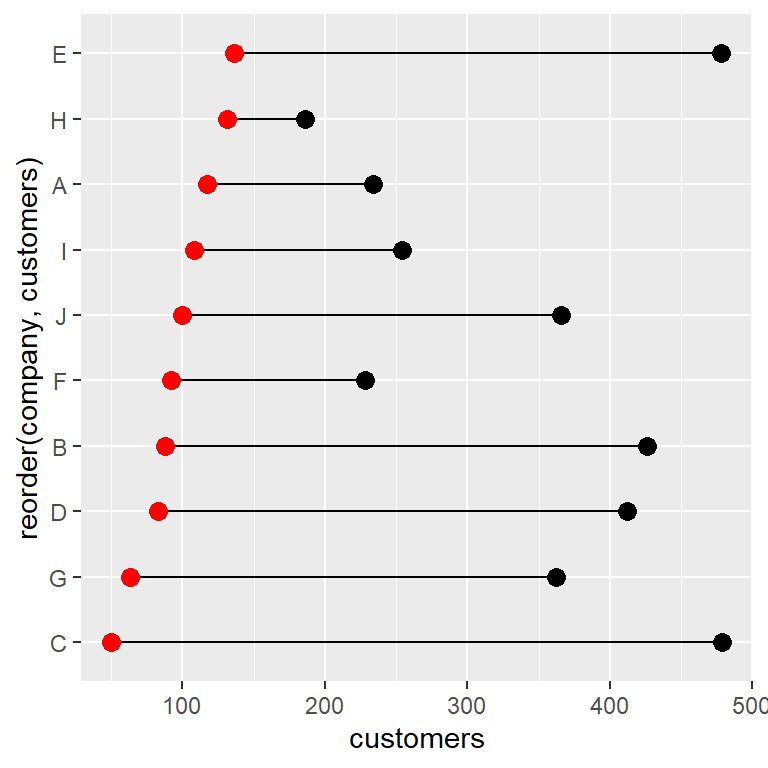Order by the mean

With the sample data frame in long format if you reorder the companies by `value` the groups will be ordered by default by its mean. Nonetheless, the `reorder` function allows specifying other functions with `FUN`, such as `min` or `max`, to reorder the values based on the minimum or maximum value, respectively.

``````# install.packages("ggplot2")
library(ggplot2)

ggplot(df2, aes(x = value, y = reorder(company, value))) +
geom_line() +
geom_point(aes(color = variable), size = 3) +
scale_color_brewer(palette = "Set1", direction = -1) +
theme(legend.position = "bottom")``````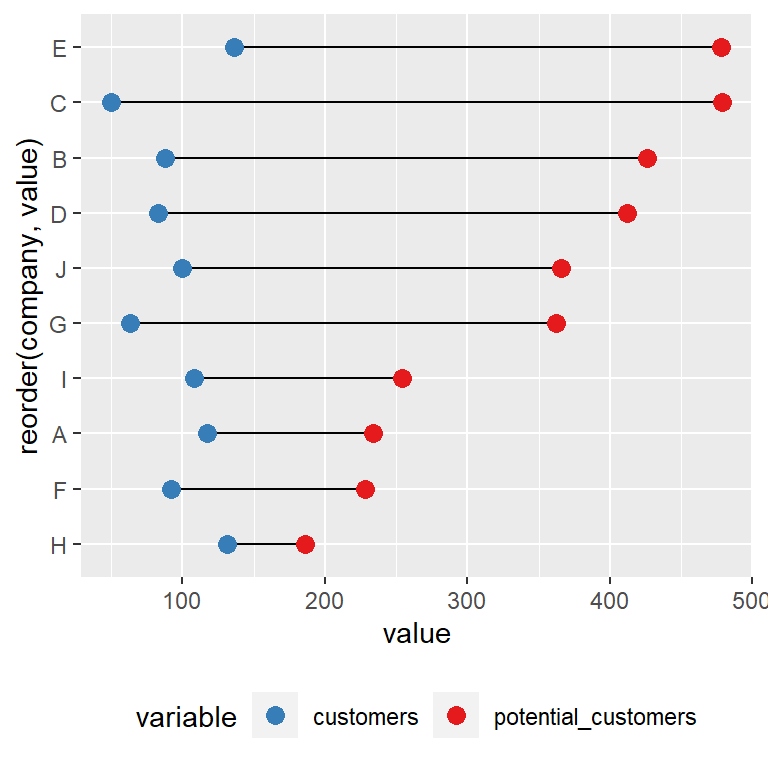Use `reorder(company, value, FUN = min)` or `reorder(company, value, FUN = max)` to reorder the data based on the minimum or máximum value for each group, respectively.

Finally, when using `geom_dumbbell`, as it works with wide format data frames the process is the same as the one used on the first example of this section. However, in the following example we are ordering the groups based on the `potential_customers` column.

``````# install.packages("ggplot2")
library(ggplot2)

ggplot(df, aes(y = reorder(company, potential_customers))) +
geom_dumbbell(aes(x = customers, xend = potential_customers),
color = "darkgray",
size = 1, dot_guide = FALSE,
size_x = 3,  size_xend = 3,
colour_x = "#F69541", colour_xend = "#699DC6")``````